# How to Divide Two Columns in SQLself.__wrap_n=self.__wrap_n||(self.CSS&&CSS.supports("text-wrap","balance")?1:2);self.__wrap_b=(e,r,t)=>{t=t||document.querySelector(`[data-br="\${e}"]`);let n=t.parentElement,a=s=>t.style.maxWidth=s+"px";t.style.maxWidth="";let o,i=n.clientWidth,l=n.clientHeight,c=i/2-.25,f=i+.5;if(i){for(a(c),c=Math.max(t.scrollWidth,c);c+1<f;)o=Math.round((c+f)/2),a(o),n.clientHeight===l?f=o:c=o;a(f*r+i*(1-r))}t.__wrap_o||typeof ResizeObserver<"u"&&(t.__wrap_o=new ResizeObserver(()=>{self.__wrap_b(0,+t.dataset.brr,t)})).observe(n)};self.__wrap_n!=1&&self.__wrap_b(":Rj0pm:",1)

November 13, 2023

In SQL, dividing two columns is a common operation used for calculating ratios or percentages. This guide shows how to perform division between two columns in an SQL query.

## Understanding basic division in SQL

To divide two columns in SQL, you use the division operator `/`. Suppose you have a table `sales_data` with columns `total_sales` and `number_of_orders`. To calculate the average sale per order, you would write:

```SELECT total_sales / number_of_orders AS average_sale_per_order
FROM sales_data;```

## Handling division by zero

Division by zero is an error in SQL. To avoid this, use the `NULLIF` function, which returns NULL if the second argument is zero, thus preventing the error:

```SELECT total_sales / NULLIF(number_of_orders, 0) AS average_sale_per_order
FROM sales_data;```

## Dividing aggregated data

When working with aggregate functions like `SUM` or `AVG`, you might want to divide the results of these aggregations. For example, to find the ratio of total sales to total orders:

```SELECT SUM(total_sales) / NULLIF(SUM(number_of_orders), 0) AS total_ratio
FROM sales_data;```

## Using division in JOIN operations

In scenarios involving JOINs, you might need to divide columns from different tables. Assuming a second table `product_data` with a `product_id` and `price`, to find the ratio of total sales to the total price of products sold:

```SELECT
SUM(s.total_sales) / NULLIF(SUM(p.price), 0) AS sales_price_ratio
FROM
sales_data s
JOIN
product_data p ON s.product_id = p.product_id;```

## Dividing columns in a subquery

Sometimes, you might need to divide columns obtained from a subquery. For instance, to calculate the ratio of two aggregates:

```SELECT
(SELECT SUM(total_sales) FROM sales_data) /
NULLIF((SELECT SUM(number_of_orders) FROM sales_data), 0) AS overall_ratio;```

## Formatting division results

To format the result of a division, especially when dealing with floating-point numbers, use the `ROUND` or `CAST` functions. For example, rounding to two decimal places:

```SELECT
ROUND(SUM(total_sales) / NULLIF(SUM(number_of_orders), 0), 2) AS formatted_ratio
FROM
sales_data;```

## Dividing columns from a grouped result

When working with grouped data, ensure that the division is done within the context of each group. For example, to calculate the average sale per order for each product:

```SELECT
product_id,
SUM(total_sales) / NULLIF(SUM(number_of_orders), 0) AS average_per_product
FROM
sales_data
GROUP BY
product_id;```

## Using Division in Case Statements

In SQL, `CASE` statements allow for conditional logic within queries. Division can be integrated into these statements to handle different scenarios dynamically. For example, to avoid division by zero or to apply different formulas under certain conditions:

```SELECT
CASE
WHEN number_of_orders > 0 THEN total_sales / number_of_orders
ELSE 0
END AS conditional_average
FROM
sales_data;```

This snippet calculates an average only if `number_of_orders` is greater than zero, otherwise it returns 0. This approach can be expanded to handle various conditions, ensuring the query remains robust and error-free.

## Dividing Across Different Data Types

SQL handles data types like integers, floats, and decimals differently, especially during division. When dividing an integer by an integer, SQL typically returns an integer. To get a decimal result, you need to convert one of the operands to a float or decimal:

```SELECT
CAST(total_sales AS FLOAT) / NULLIF(number_of_orders, 0) AS average_sale_per_order
FROM
sales_data;```

Here, `CAST(total_sales AS FLOAT)` ensures that the division results in a float, even if `total_sales` and `number_of_orders` are integers. Understanding and correctly handling these data type conversions is crucial for accurate results, especially in financial or scientific computations where precision matters.

### Dividing Columns Across Multiple Joined Tables

When dealing with multiple tables, division can become more complex. For example, if you need to divide values from columns located in different tables, you’ll typically use a JOIN:

```SELECT
s.product_id,
SUM(s.total_sales) / NULLIF(SUM(p.quantity_sold), 0) AS sales_to_quantity_ratio
FROM
sales_data s
JOIN
product_data p ON s.product_id = p.product_id
GROUP BY
s.product_id;```

This query calculates the ratio of `total_sales` to `quantity_sold` for each product, combining data from `sales_data` and `product_data`.

### Using Division in Window Functions

SQL window functions allow for complex calculations across sets of rows related to the current row. For example, to calculate a running average sale per order:

```SELECT
product_id,
total_sales,
number_of_orders,
AVG(total_sales) OVER (ORDER BY order_date) / NULLIF(AVG(number_of_orders) OVER (ORDER BY order_date), 0) AS running_average
FROM
sales_data;```

This query calculates a running average of sales per order, ordered by `order_date`. Window functions are powerful tools for performing sophisticated analytical queries in SQL.

## Conclusion

Dividing columns in SQL is a straightforward process, but it requires careful handling of cases like division by zero. Using functions like `NULLIF` and `ROUND`, you can ensure accurate and meaningful results from your SQL queries.

# Basedash is the best MySQL admin panel

If you're building with MySQL, you need Basedash. It gives you an instantly generated admin panel to understand, query, build dashboards, edit, and share access to your data.

If you're building with MySQL, you need Basedash. It gives you an instantly generated admin panel to understand, query, build dashboards, edit, and share access to your data.

If you're building with MySQL, you need Basedash. It gives you an instantly generated admin panel to understand, query, build dashboards, edit, and share access to your data.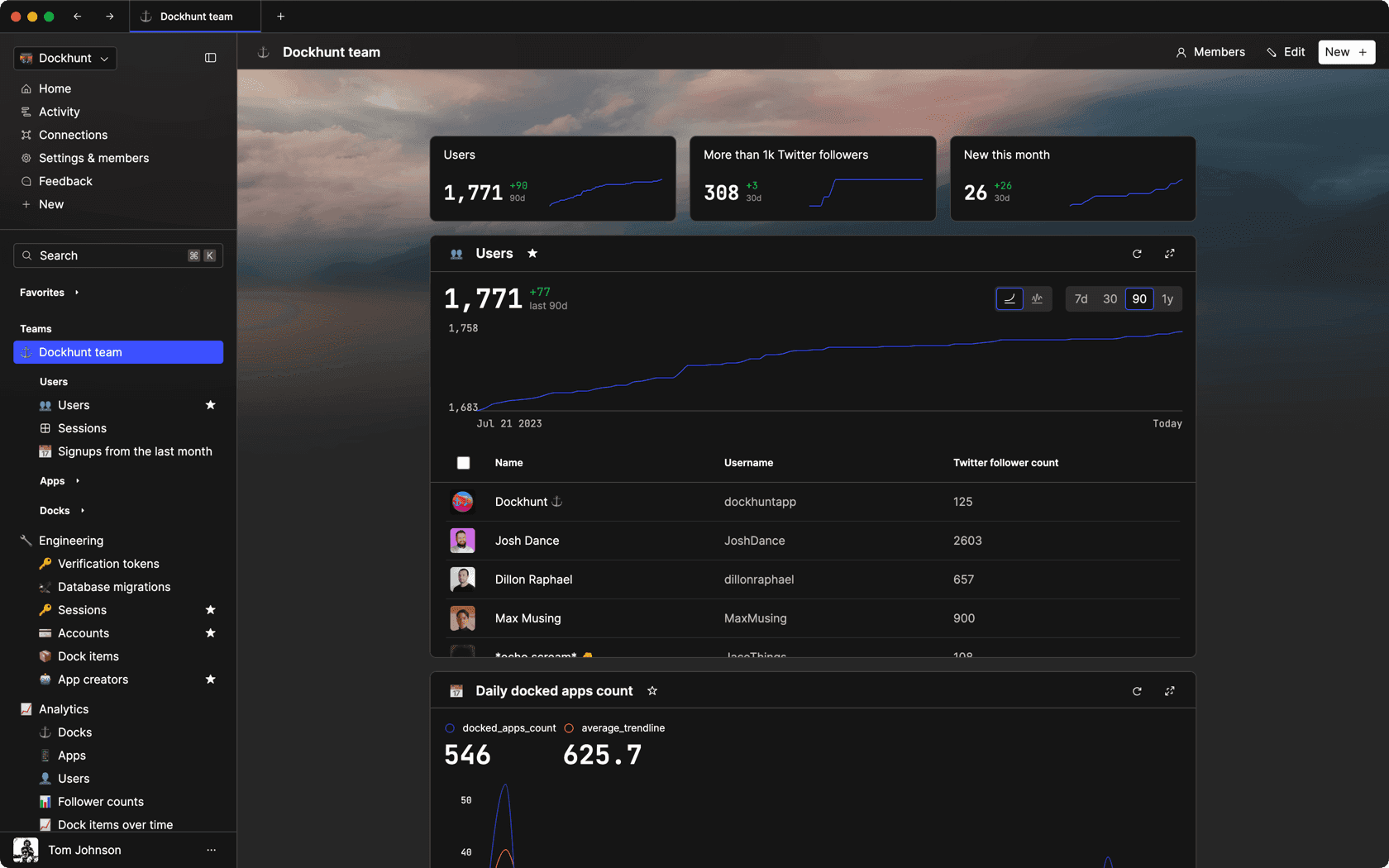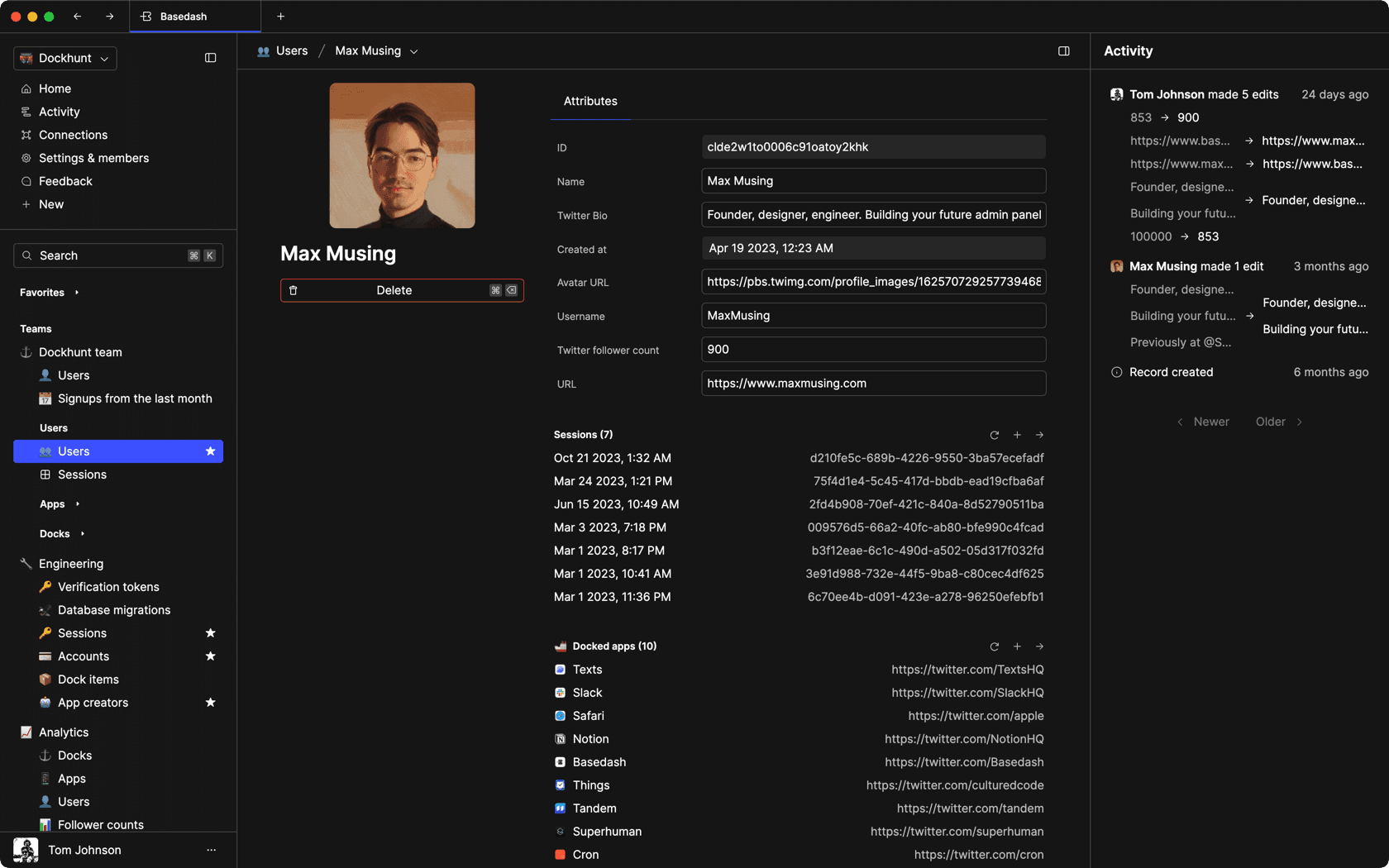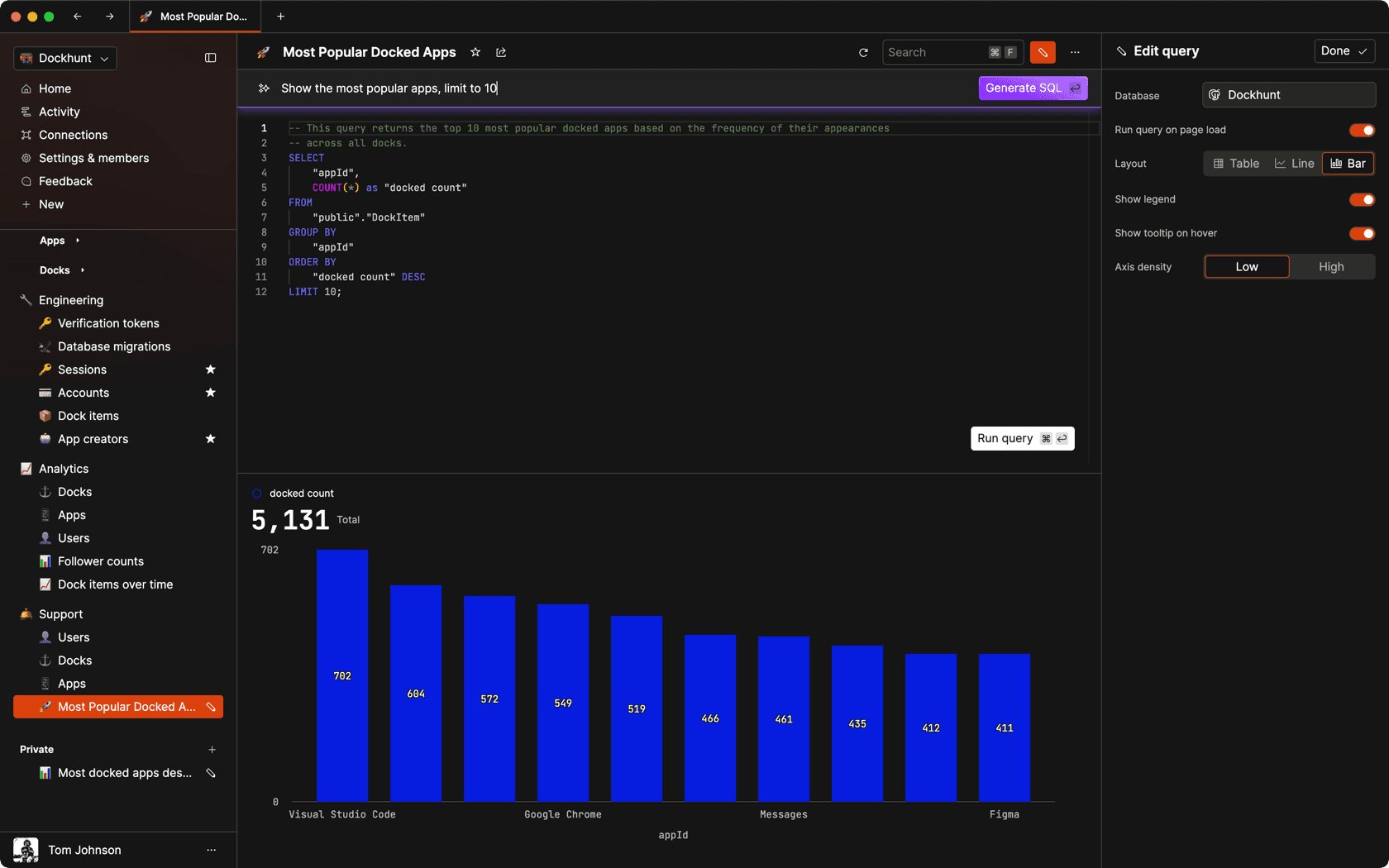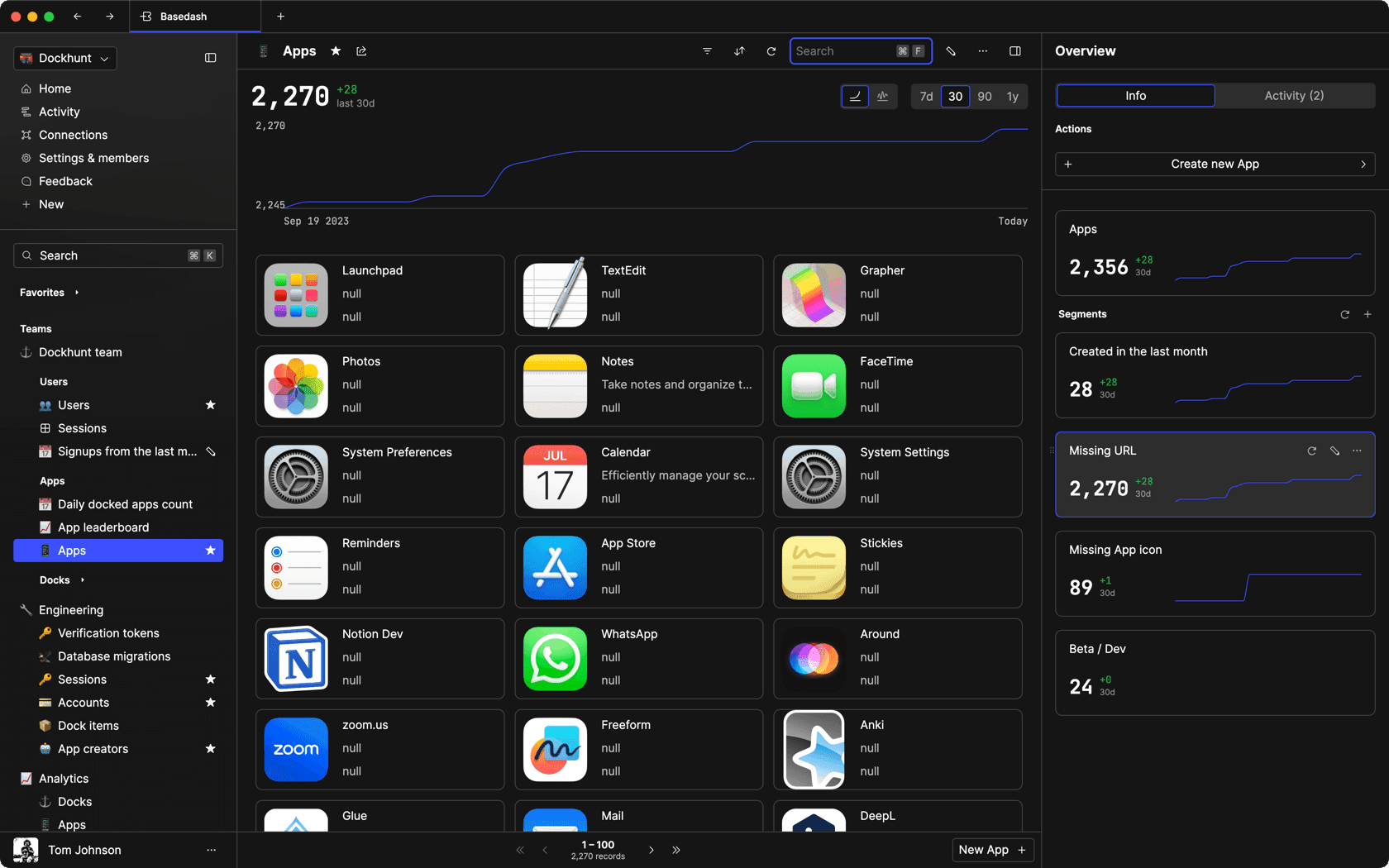### Dashboards and charts

Effortlessly make charts and create a space for your team to work together towards shared goals and metrics.

### SQL composer with AI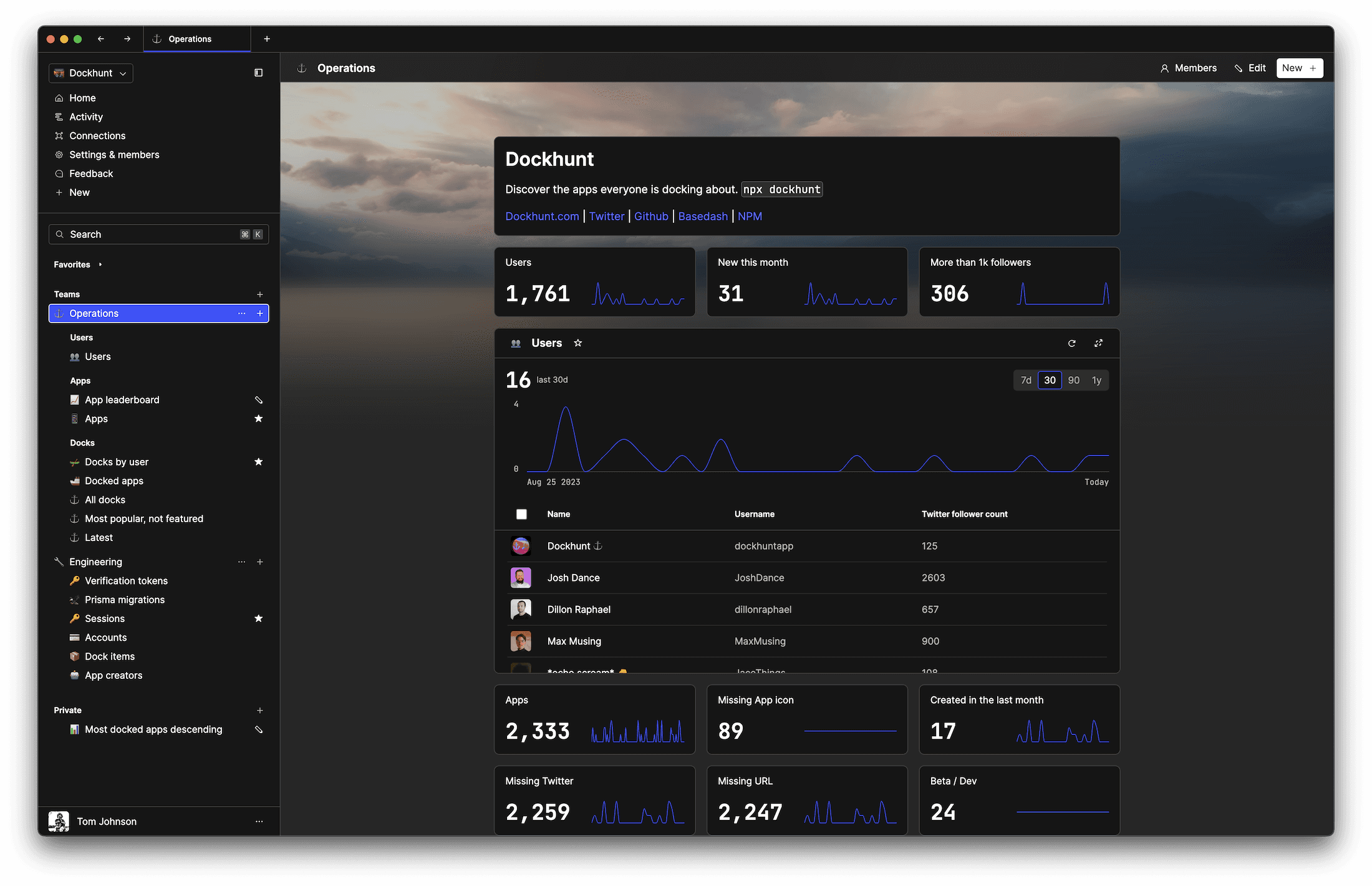SOC II
Type 2 Certified

### Features

Proudly carbon-neutral.SOC II
Type 2 Certified

### Features

Proudly carbon-neutral.SOC II
Type 2 Certified